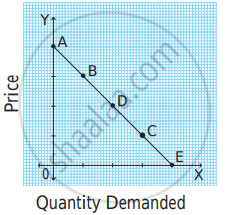# In the following diagram, AE is the linear demand curve of a commodity. On the basis of the given diagram state whether the following statement is True or False. - Economics

MCQ
True or False

In the following diagram, AE is the linear demand curve of a commodity. On the basis of the given diagram state whether the following statement is True or False. Give a reason for your answer.Demand at point ‘D’ is perfectly inelastic demand.

• True

• False

#### Solution

False.

Correct Statement: Demand at point ‘D’ is unitary elastic demand.

Reason: The length of the lower segment is equal to the upper segment at point D. Therefore, Ed = 1. Thus, at point D, demand is unitary elastic.

Concept: Types of Price Elasticity of Demand
Is there an error in this question or solution?

#### APPEARS IN

Balbharati Economics 12th Standard HSC Maharashtra State Board
Chapter 3.2 Elasticity of Demand
Exercise | Q 7. (2)(3) | Page 36